Maths-
General
Easy

Question

# Calculate the area and height of equilateral triangle whose perimeter is 48 cmHint:

## The correct answer is: 13.86 cm

### Let side of the triangle be aIt is given that perimeter of an equilateral triangle = 48⇒ 3a = 48⇒ a = 16 cmArea of an equilateral triangle =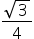(side)2=(16)2  = 64 cm2 (Substituting the value of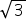= 1.73, in the above equation)= 110.85 cm2Area of triangle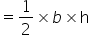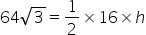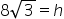So, height of the triangle =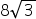= 13.86 cm#### With Turito Foundation.#### Get an Expert Advice From Turito.